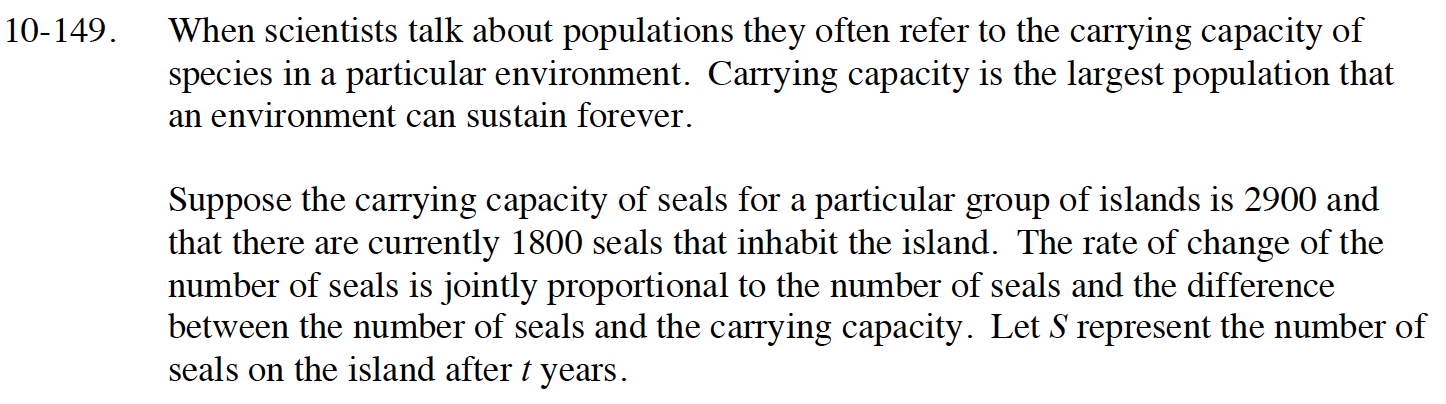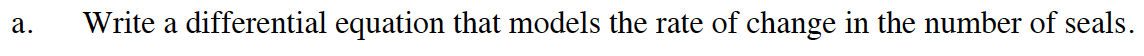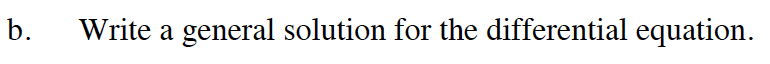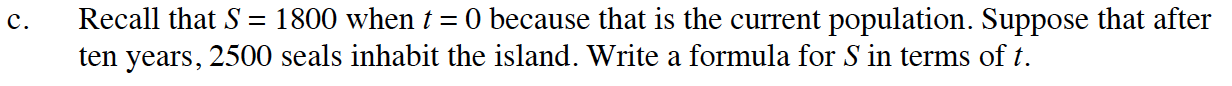### Home > CALC > Chapter Ch10 > Lesson 10.4.2 > Problem10-149

10-149.
1. When scientists talk about populations they often refer to the carrying capacity of species in a particular environment. Carrying capacity is the largest population that an environment can sustain forever.

Suppose the carrying capacity of seals for a particular group of islands is 2900 and that there are currently 1800 seals that inhabit the island. The rate of change of the number of seals is jointly proportional to the number of seals and the difference between the number of seals and the carrying capacity. Let S represent the number of seals on the island after t years. Homework Help ✎

1. Write a differential equation that models the rate of change in the number of seals.

2. Find a general solution to the differential equation.

3. Recall that S = 1800 when t = 0 because that is the current population. Suppose that after ten years, 2500 seals inhabit the island. Write a formula for S in terms of t.$\frac{dS}{dt}=kS(2900-S)$$\frac{dS}{S(2900-S)}=kdt$

$\frac{1}{S(2900-S)}=\frac{1/2900}{S}+\frac{1/2900}{2900-S}$

$\ln\Big|\frac{S}{2900-S}\Big|=2900kt+C$$S=\frac{2900\Big(\frac{18}{11}\Big)e^{2900kt}}{1+\frac{18}{11}e^{2900kt}}$